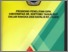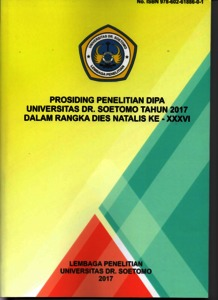# DETERMINAN BELANJA MODAL DAN PENGARUHNYA TERHADAP PENDAPATAN PERKAPITA DI PROVINSI JAWA TIMUR

Suprijati, Jajuk and Ratna Damayanti, Shanty DETERMINAN BELANJA MODAL DAN PENGARUHNYA TERHADAP PENDAPATAN PERKAPITA DI PROVINSI JAWA TIMUR. In: PROSIDING DIPA UNIVERSITAS DR. SOETOMO TAHUN 2017 DALAM RANGKA DIES NATALIS KE - XXXVI. Lembaga Penelitian Universitas Dr. Soetomo Surabaya, pp. 119-131. ISBN 978-602-61886-0-1Preview
Text
Untitled.pdf

Download (1MB) | Preview

## Abstract

This study aims to examine the factors affecting Capital Expenditure and then test the influence of these factors and Capital Expenditure on Income per capita in East Java. Factors affecting capital expenditures are X1 Local Income (PAD) X1, General Allocation Fund (DAU) X2, Special Allocation Fund (DAK) X3 and Financial Assistance (BK) X4, which then These factors become independent variables. While the dependent variable consists of Capital Expenditure (BM) Y1 and Income Perkapita (PP) Y2 .. The sample in the study consisted of 29 districts and 9 cities in East Java Province with a research period of seven years ie budget year 2003 - 2015. Sampling technique Selected by purposive sampling. The data used are secondary data taken from APBD Realization report of fiscal year 2003 - 2015 and data derived from BPS. This research method uses Analyst Model Path or precisely Two Path Analysis Model because it uses two dependent variable and four independent variables. The results of this study indicate that: From the processing of data of phase I obtained in table coefficient can be seen that the value of significance of four variables that is X1 = 0,000 and X3 = 0.000 positive significantly smaller than 0,005 and X2 = 0,004 and X4 = 0,000 negative significant less than 0,05. This result gives the conclusion that Regression of Model I that variabal X1, X2, X3 and X4 have significant effect to variable Y1, and in this model I free vaiabel does not influence simultaneously on variable Y1. The magnitude value of R2 equal to 0,919 this indicate contribution or contribution influence X1, X2, X3 and X4 to Y is 91.9% while the remaining 8.1% is the contribution of other variables not included in the research, From the processing of data of phase II obtained in the table coefficient can be seen that the significance value of four variables that is X1 = 0,000 positive significant and X2 = 0,000 significant negative and X4 = 0.011 negative significantly smaller than 0.05 medium X3 = 0.368 and Y1 = 0.660 not significant . This result gives the conclusion that Regression of Model II that is variabal X1, X2, X4 have significant effect to variable Y2.Pada model II this free variable does not have simultaneous effect on variable Y2 R2 value equal to 0,546 this indicate contribution or contribution influence X1, X2, and X4 to Y2 is 54.6% while the remaining 45.2% is the contribution of other variables not included in the researcher

Item Type: Book Section H Social Sciences > HB Economic TheoryH Social Sciences > HG FinanceL Education > L Education (General) Fakultas Ekonomi dan Bisnis > Studi Pembangunan Aditya Masruri 18 Jul 2017 02:25 18 Jul 2017 02:25 http://repository.unitomo.ac.id/id/eprint/477

### Actions (login required)View Item